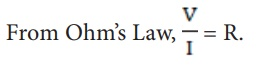Home | | Science 10th Std | Resistance of a Material

# Resistance of a Material

The resistance of a conductor can be defined as the ratio between the potential difference across the ends of the conductor and the current flowing through it.

RESISTANCE OF A MATERIAL

In Figure 4.4, a Nichrome wire was connected between X and Y. If you replace the Nichrome wire with a copper wire and conduct the same experiment, you will notice a different current for the same value of the potential difference across the wire. If you again replace the copper wire with an aluminium wire, you will get another value for the current passing through it. From equation (4.3), you have learnt that V/I must be equal to the resistance of the conductor used. The variations in the current for the same values of potential difference indicate that the resistance of different materials is different. Now, the primary question is, â€śwhat is resistance?â€ťResistance of a material is its property to oppose the flow of charges and hence the passage of current through it. It is different for different materials.

From Ohmâ€™s Law, V / I = R.The resistance of a conductor can be defined as the ratio between the potential difference across the ends of the conductor and the current flowing through it.

## Unit of Resistance

The SI unit of resistance is ohm and it is represented by the symbol â„¦.

Resistance of a conductor is said to be one ohm if a current of one ampere flows through it when a potential difference of one volt is maintained across its ends.

1 ohm = 1 volt / 1 ampere### Solved Problem

Calculate the resistance of a conductor through which a current of 2 A passes, when the potential difference between its ends is 30 V.

### Solution:

Current through the conductor I = 2 A,

Potential Difference V = 30 V

From Ohmâ€™s Law: R = V/I.

Therefore, R = 30/2 = 15 â„¦

Study Material, Lecturing Notes, Assignment, Reference, Wiki description explanation, brief detail
10th Science : Chapter 4 : Electricity : Resistance of a Material |